Reach Us+44-7482-875032
Convergence Control Parameter Region for an Unsteady Three Dimensional Navier-Stokes Equations of Flow between Two Parallel Disks by using Homotopy Analysis Method
Journal of Applied & Computational Mathematics
All submissions of the EM system will be redirected to Online Manuscript Submission System. Authors are requested to submit articles directly to Online Manuscript Submission System of respective journal.

# Convergence Control Parameter Region for an Unsteady Three Dimensional Navier-Stokes Equations of Flow between Two Parallel Disks by using Homotopy Analysis Method

Selvarani S* and Beulah RD

Department of Mathematics, VLB Janakiammal College of Arts and Science, Coimbatore-641 042, Tamilnadu, India

*Corresponding Author:
Selvarani S
Department of Mathematics,
VLB Janakiammal College of Arts and Science
Coimbatore - 641 042, Tamilnadu, India
Tel: 0422260779
E-mail: [email protected]

Received: November 20, 2015; Accepted: December 11, 2015; Published: December 17, 2015

Citation: Selvarani S, Beulah RD (2015) Convergence Control Parameter Region for an Unsteady Three Dimensional Navier-Stokes Equations of Flow between Two Parallel Disks by using Homotopy Analysis Method. J Appl Computat Math 4:277. doi:10.4172/2168-9679.1000277

Copyright: © 2015 Selvarani S, et al. This is an open-access article distributed under the terms of the Creative Commons Attribution License, which permits unrestricted use, distribution, and reproduction in any medium, provided the original author and source are credited.

Visit for more related articles at Journal of Applied & Computational Mathematics

#### Abstract

Purpose: The paper aims to find the convergence control parameter region for an unsteady three dimensional Navier-Stokes equations of flow between two parallel disks by using Homotopy Analysis Method. Findings: The region and value of the convergence control parameter has been found.

#### Keywords

3D Navier-Stokes equation; Homotopy analysis method; Convergence; System of nonlinear differential eqiations

#### Introduction

In mathematics and physics, nonlinear partial differential equations are partial differential equations with nonlinear terms. A few nonlinear differential equations have known exact solutions, but many which are important in applications do not. Sometimes these equations may be linearized by an expansion process in which nonlinear terms are discarded. When nonlinear terms make vital contributions to the solution this cannot be done, but sometimes it is enough to retain a few small ones. Then a perturbation theory may be used to obtain the solution. The differential equations may sometimes be approximated by an equation with small nonlinearities in more than one way, giving rise to different solutions valid over different range of its parameters.

Most scientific and engineering problems are modeled by ordinary differential equations or partial differential equations, Some of them are solved using the analytic methods of perturbation by Nayfeh . In the numerical methods, stability and convergence should be considered so as to avoid divergence or inappropriate results. In the analytic perturbation methods, we should excert the small parameter in the equation. In numerical methods the advantage is that we have to use the small parameter a lot since most problems do not have known analytic solutions, or that if they are known it is too complex to deal with them. The main advantage in analytic method is that it is exact and gives us more context. One of the semi-exact methods which do not need small or large parameters is the Homotopy Analysis Method (HAM), first proposed by Liao in his Ph.D thesis. Liao  employed the basic ideas of homotopy in topology to propose a general analytic method for nonlinear problems, namely HAM, which is a powerful analytical method for solving linear and nonlinear differential equations. The HAM also avoids discretization and provides an efficient solution with high accuracy, minimal calculations and avoidance of physically unrealistic assumption. Furthermore, the HAM always provides us with a family of solution expressions with the auxiliary parameter h, the convergence region and the rate of each solution might be determined conveniently by the auxiliary parameter h. HAM contains the homotopy perturbation method (HPM) discussed by He , the Adomian decomposition method (ADM) examined by Allan , and the d-expansion method.

The main goal of the present study is to find the value of convergence control parameter for the problem of flow between two disks by the HAM.

#### Mathematical Formulation

Consider the axis-symmetric flow between two infinite disks with a distance d between them. Both disks are placed in the radial direction with a velocity proportional to the radii. The bottom disk is located in the z = 0 plane. The velocity ratio of the upper disk to the lower one is γ and ε is the amplitude of the disk. For an incompressible fluid without body forces and based on axis symmetric reads from the papers discussed by Dinarvand  and Munnavar .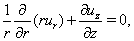(1)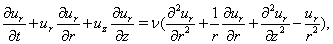(2)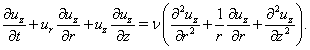(3)

Where the velocity vector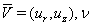is the kinematic viscosity. By using von Karman type similarity transformations, similarity functions can be sought as follows,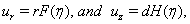where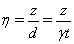is the similarity variable. Substituting the similarity functions into the equations (1), (2) and (3). Therefore, the governing equations yields a similarity equation group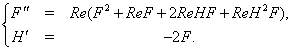(4)

with boundary conditions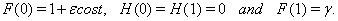(5)

Where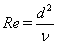is the Reynolds number of the wall and γ is the parameter of the upper disk showing the velocity ratio of the upper disk to the bottom disk. Without loss of generality, we assumed that 0 ≤ γ ≤ 1.

Analytical solution with HAM

Due to basic idea of HAM, as described in detail by Liao [7,8], according to the boundary conditions (5), we choose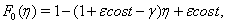(6)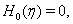(7)

as initial guesses of F(η), and H(η) which satisfy the boundary conditions (5). Besides, we select the auxiliary linear operators L1(F), and L2(H) as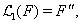(8)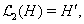(9)

satisfying the follwing properties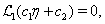(10)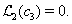(11)

Where ci, I=1, 2, 3 are arbitrary constants. If q ∈ [0, 1] is an embedding parameter and h is an auxiliary nonzero parameter, then the zeroth-order deformation equations are of the following form,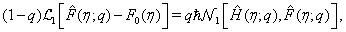(12)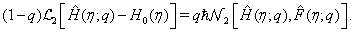(13)

subject to the boundary conditions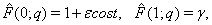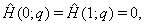in which we define the nonlinear operators N1 and N2 as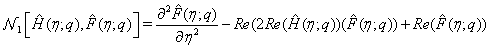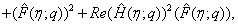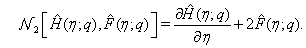Clearly, when q = 0 the zero-order deformation equations (12) and (13) give rise to: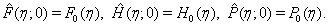(14)

when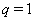, they become: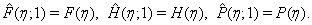(15)

As q increases from 0 to 1,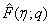and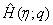vary from F0(η) and H0(η) to F(η) and H(η).

Expanding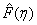and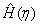in Maclaurin series with respect to the embedding parameter q and equations (14) and (15), we obtain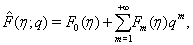(16)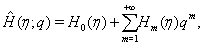(17)

where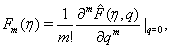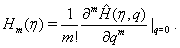As pointed by Liao , the convergence of the series (16) – (17) strongly depend upon auxiliary parameter h. Assume that h is selected such that the series (16) – (17) are convergent at q = 1 then due to equations (14) and (15) we have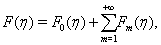(18)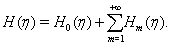(19)

Differentiating the zero-order deformation equations (12) and (13) m times with respect to q, then setting q = 0 and finally dividing by m! we have the mth - order deformation equations [10-18].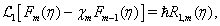(20)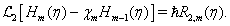(21)

with the following boundary conditions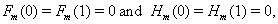(22)

where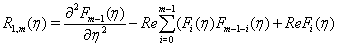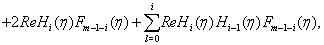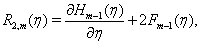and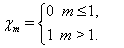Then the solutions for equations (20) and (21) can be expressed by: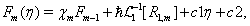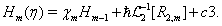Where c1, c2, c3 are integral constants can be found by boundary conditions (22). For example, we can obtain the following result for solving the first-order deformation equation by using symbolic software MATHEMATICA, and successively obtain [19-25],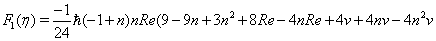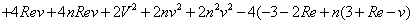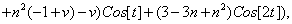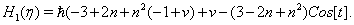Convergence of HAM solution

The totally analytic series solutions of the functions F(η) and H(η) are given in equations (18) – (1819). The convergence of these series and the rate of approximation for the HAM strongly depends upon the value of the auxiliary parameter h, as pointed out by Liao . In general, by means of the h-curve, it is straightforward to choose a proper value of h to control the convergence of the approximation series. To find the range of the admissible values of h, h - curves of F″(0) and H′(0) obtained by the 11th order approximation of the HAM for γ = 0 and γ = 1 at Re = 1, t = 1 and ε = 1 are plotted in Figures 1 and 2, respectively. From these figures, the valid regions of h correspond to the line segments nearly parallel to the horizontal axis. Sometimes this region is not perfectly flat to the slowly convergence rate of the series solution which was discussed by Liao in his book Homotopy Analysis Method. However a value of h can be picked up. Therefore in this problem we can choose h = -0.4 [26-28].

Figure 1: The h – curve of F”(0), obtained by 11th order approximation of the HAM for γ=0, γ=1, ε=1 and t=1 with Re=1.

Figure 2: The h – curve of H”(0), obtained by 11th order approximation of the HAM for γ=0, γ=1, ε=1 and t=1 with Re=1.

#### Conclusion

In this paper, the HAM was used for finding the convergence control parameter of the system of nonlinear ODE derived from von Karman type similarity transform for the unsteady state three dimensional Navier-Stokes equations of flow between two parallel disks. Unlike perturbation methods, the HAM does not depend on any small physical parameters. Thus homotopy analysis method is valid for both weakly and strongly nonlinear problems. Different from all other analytic methods, the homotopy analysis method provides us a simple way to adjust and control the convergence region of the series solution by means of auxiliary parameter h. Thus, the auxiliary parameter h plays a vital role within the frame of HAM which can be determined by the h curves.

#### References

Select your language of interest to view the total content in your interested language

### Article Usage

• Total views: 8414
• [From(publication date):
December-2015 - Aug 21, 2019]
• Breakdown by view type
• HTML page views : 8306
• PDF downloads : 108

## Post your commentCan't read the image? click here to refresh
###### Peer Reviewed Journals

Make the best use of Scientific Research and information from our 700 + peer reviewed, Open Access Journals

International Conferences 2019-20

Meet Inspiring Speakers and Experts at our 3000+ Global Annual Meetings

Top